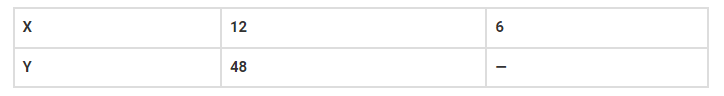# If x varies directly as y,

Question:

If x varies directly as y, thenSolution:

If x and y varies directly, then;

x/y = k

If x=12 and y = 48, then

k = 12/48 = ¼

Now, if x=6, and k = 1/4, then y will be;

6/y = ¼

y = 6×4=24